# Difference between revisions of "Mean spherical approximation"

The Lebowitz and Percus mean spherical approximation (MSA) (1966) (Ref. 1) closure relation is given by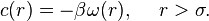$c(r) = -\beta \omega(r), ~~~~ r>\sigma.$

In the Blum and Høye mean spherical approximation for mixtures (Refs 2 and 3) the closure is given by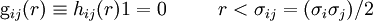${\rm g}_{ij}(r) \equiv h_{ij}(r) 1=0 ~~~~~~~~ r < \sigma_{ij} = (\sigma_i \sigma_j)/2$

and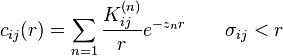$c_{ij}(r)= \sum_{n=1} \frac{K_{ij}^{(n)}}{r}e^{-z_nr} ~~~~~~ \sigma_{ij} < r$

where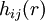$h_{ij}(r)$ and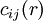$c_{ij}(r)$ are the total and the direct correlation functions for two spherical molecules of i and j species,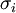$\sigma_i$ is the diameter of 'i species of molecule. Duh and Haymet (Eq. 9 Ref. 4) write the MSA approximation as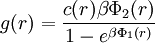$g(r) = \frac{c(r) \beta \Phi_2(r)}{1-e^{\beta \Phi_1(r)}}$

where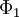$\Phi_1$ and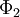$\Phi_2$ comes from the Weeks-Chandler-Anderson division of the Lennard-Jones potential. By introducing the definition (Eq. 10 Ref. 4)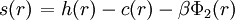$\left.s(r)\right. = h(r) -c(r) -\beta \Phi_2 (r)$

one can arrive at (Eq. 11 in Ref. 4)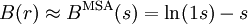$B(r) \approx B^{\rm MSA}(s) = \ln (1 s)-s$

The Percus Yevick approximation may be recovered from the above equation by setting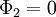$\Phi_2=0$.

See Ref. 5.